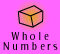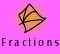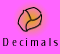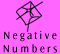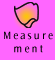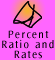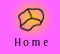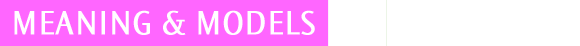| When do we use negative numbers? | What are directed numbers? |
| Models for directed number arithmetic |
| Quick quiz |

When do we use negative numbers?

We use negative numbers in our everyday life without thinking about it.

'It was so cold last night, the temperature was 4 degrees below zero!'

Another way of saying '4 degrees below zero' is -4 ° C.

'Lake Eyre is 16 metres below sea level.'

We refer to sea level as the zero point for measuring landforms, such as mountains, so on this scale the height of Lake Eyre is actually -16 metres.

'My bank account is in the red by \$150!'

'In the red' is a common phrase used when we talk about banking, meaning that we owe money. If I am 'in the red' by \$150 I actually have -\$150 in my account. (This means the bank has lent me \$150 and eventually I will need to pay it back.)

'Jerry made a loss on the horses of \$20.'

If Jerry made a loss of \$20 on the horses, this means he has \$20 less than before he started betting.

What are Directed Numbers?

In each of the above cases we can think of opposite examples: The temperature can be either warmer or cooler than zero. A mountain or a lake can be above or below sea level. A bank account can be in credit or debit.

For every positive number there is an opposite negative number. For example, the opposite of 4 is -4 and the opposite of -239 is 239. This concept is illustrated well on a number line, see below in models for directed number arithmetic.

Negative and positive numbers are useful to indicate direction either side of a zero reference point, the positive numbers indicate one direction and the negative numbers indicate the opposite direction. Because negative and positive numbers give direction they are often called directed numbers.

While the term negative numbers is commonly used, in many cases the term directed numbers would be more appropriate. For example, if we are talking about 'negative number arithmetic' we often mean this to include arithmetic of both negative and positive numbers, i.e. directed numbers, therefore it is useful to be aware that the terms negative numbers and directed numbers are often used interchangeably. When we are only working with positive numbers we usually just refer to them as numbers.

When we use directed numbers we are often referring to real contexts where opposites are involved. Can you think of others?

 positive (+) negative (-) above below right left up down forward backward profit loss increase decrease anti-clockwise clockwise

Models for directed number arithmetic

There are a number of models which can be used to illustrate operations with negative and positive numbers. In this topic we will be mainly using integers, by this we mean whole numbers and the negatives of whole numbers. However we need to be aware that there are many other negative numbers other than the negatives of whole numbers, for example, -1.5, -1/2 and -3.45321. These negative numbers can be represented on the number line also, as can their opposites of 1.5, 1/2 and 3.45321, and follow exactly the same mathematical rules as negative integers. The only reason we are talking about integers here is because they are the simplest for a teaching context.

 Definition: Integers consist of all whole numbers and the negatives of these.

The number line model
We have all seen the number line used to show that there are numbers positioned in both directions from zero. The numbers to the right of zero are positive and the numbers to the left of zero are negative. Zero is neither negative nor positive.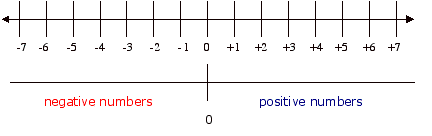If we pick any number on the number line all the numbers to the left of it are smaller. We can also see that all numbers to the right of it are bigger. This model represents a number by a point on a line.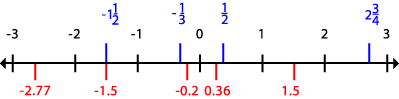A number line showing integers, decimals and fractions

The Annihilation model

A model which is useful for illustrating operations involving positive and negative numbers is the annihilation model. In this model a number is represented by a collection of chips. The annihilation model has positive chips and negative chips and works on the basis that a positive chip will annihilate a negative chip and vice versa. Sometimes the positive chip is black and sometimes the negative chip is red in analogy with colours used for credit and debit.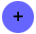a positive chip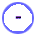a negative chip

The table below shows the model in use. The positive and negative chips can be arranged to represent positive or negative integers.

 Collection Model Number Represented Thinking +1, +1, +1+3 Three positive chips represent +3. -1, -1-2 Two negative chips represent -2. -1, +1, -1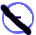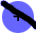-1 Two +1s and one -1 represent -1, since one negative chip and one positive chip annihilate each other, leaving one negative. +1, +1, +1, +1, -1, -1, -1, -1, -1-1 Four positive chips and five negative chips also represent -1, since four positive chips annihilate four negative chips, leaving one negative.

Note that any number can be represented in many ways.

Which model is best?
Both these models represent numbers well, although they demonstrate different properties best. For example, the number line is very good for demonstrating the ordering of numbers. The annihilation model represents integers very well. Both models demonstrate some of the operations on integers but not all.
This is discussed in more detail in the Operations, Multiplying Negative Numbers section.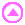Quick quiz

 1. Write each of the following as directed numbers: a) 150 metres below sea level b) a fine of \$75 c) a temperature drop of 8 degrees d) 1973 metres above sea level e) a lead of 25 metres f) a drop of 30 centimetres g) a loan of \$1500 h) a profit of \$300 2. a) If the temperature in the city today was 11 degrees and it dropped by 5 degrees overnight, what was the minimum temperature overnight? b) If the temperature was only 8 degrees in The Dandenongs during the day and dropped by 10 degrees overnight, what did it drop to? c) If the temperature at Mt Hotham last night was -3 degrees and it has risen to 7 degrees today, by how much did it rise? 3. Clara and Than started their climb at the bottom of a dry lake bed at 20 metres below sea level. a) If Clara and Than climbed 100 metres, how high above sea level did they climb? b) If Clara and Than climbed a further 450 metres, how high above sea level are they now?